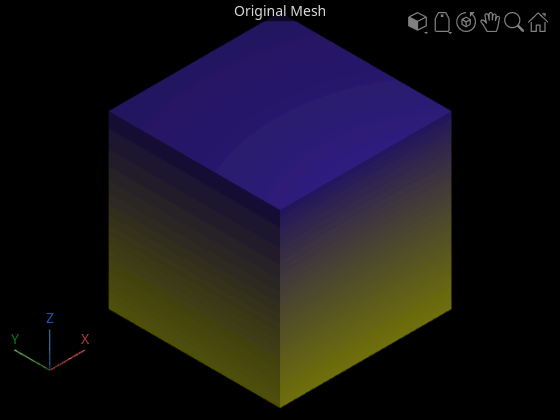# subdivide

Subdivide surface mesh

Since R2022b

## Syntax

``subdivide(mesh,"midpoint-split",numIterations)``
``subdivide(mesh,"loop",numIterations)``

## Description

example

````subdivide(mesh,"midpoint-split",numIterations)` subdivides the surface mesh `mesh` by using the midpoint-split method with the specified number of iterations. In this method, the function divides each face of the mesh into four faces in each iteration. New vertices lie at the midpoints of the edges of the original face.```

example

````subdivide(mesh,"loop",numIterations)` subdivides the surface mesh by using a loop subdivision method with the specified number of iterations. In this methods, the function divides each triangular face into four by connecting the midpoints of the edges, then updates the new vertices as a weighted average of neighboring positions. The function divides each face into four faces in each iteration. ```

## Examples

collapse all

Define mesh vertices for a surface mesh.

```vertices = [1 -1 1; 1 1 1; -1 1 1; -1 -1 1; ... 1 -1 -1; 1 1 -1; -1 1 -1; -1 -1 -1];```

Define the mesh faces using the vertices.

```faces = [6 2 1; 1 5 6; 8 4 3; 3 7 8; 6 7 3; 3 2 6; ... 5 1 4; 4 8 5; 4 1 2; 2 3 4; 7 6 5; 5 8 7];```

Create and display the surface mesh.

```mesh = surfaceMesh(vertices,faces); surfaceMeshShow(mesh,Title="Original Mesh")```Subdivide the mesh using the midpoint-split method. Display the subdivided mesh.

```numIterations = 4; subdivide(mesh,"midpoint-split",numIterations); surfaceMeshShow(mesh,Title="Subdivided Mesh",WireFrame=true)```Read a surface mesh from a PLY file.

```fileName = fullfile(toolboxdir("lidar"),"lidardata", ... "surfaceMesh","sphere.ply"); mesh = readSurfaceMesh(fileName);```

Display the surface mesh.

`surfaceMeshShow(mesh,Title="Original Mesh",WireFrame=true)`Subdivide the mesh using the loop method, and display the result.

```numIterations = 1; subdivide(mesh,"loop",numIterations) surfaceMeshShow(mesh,Title="Subdivided mesh",WireFrame=true)```## Input Arguments

collapse all

Surface mesh, specified as a `surfaceMesh` object.

Number of iterations for the subdivision method, specified as a positive integer. At each iteration, the function divides each face of the mesh into four faces.

Data Types: `single` | `double` | `int8` | `int16` | `int32` | `int64` | `uint8` | `uint16` | `uint32` | `uint64`

## Limitations

The function discards the `FaceColors` property of the input `surfaceMesh` object. The subdivided mesh does not have any face colors.

## Version History

Introduced in R2022b

expand all